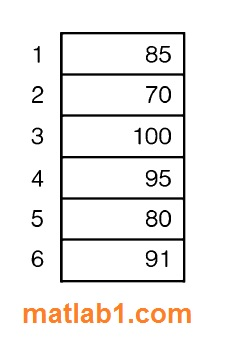Sorting is the process of putting a list in order-either descending (highest to lowest) or ascending (lowest to highest) order. For example, here is a list of n integers, visualized as a column vector.What is desired is to sort this in ascending order in place-by rearranging this vector, not creating another. The following is one basic algorithm.
• Look through the vector to find the smallest number and then put it in the first element in the vector. How? By exchanging it with the number currently in the first element.
• Then, scan the rest of the vector (from the second element down) looking for the next smallest (or, the smallest in the rest of the vector). When found, put it in the first element of the rest of the vector (again, by exchanging).
• Continue doing this for the rest of the vector. Once the next-to-last number has been placed in the correct location in the vector, the last number, by default, has been as well.

What is important in each pass through the vector is where the smallest value is so the elements to be exchanged are known (not what the actual smallest number is).
This table shows the progression. The left column shows the original vector. The second column (from the left) shows that the smallest number, the 70, is now in the first element in the vector. It was put there by exchanging with what had been in the first element, 85. This continues element-by-element, until the vector has been sorted.This is called the selection sort; it is one of many different sorting algorithms.

Sorting a row vector results in another row vector. Sorting a column vector results in another column vector. Note that if we did not have the’ descend’ option, fliplr (for a row vector) or flipud (for a column vector) could be used after sorting.

For matrices, the sort function will by default sort each column. To sort by rows, the dimension 2 is specified. For example,

```>> mat
mat=
4    6    2
8    3    7
9    7    1```
```>> sort (mat) % sorts by column
ans=```
```4    3    1
8    6    2
9    7    7
>> sort(mat,2) %sorts by row
ans=```
```2    4    2
3    7    8
1    7    9```